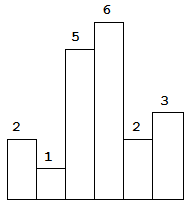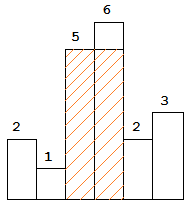# 「力扣」第 84 题：柱状图中最大的矩形（困难）

### 题目描述输入: [2,1,5,6,2,3]

### 方法一：暴力解法

1、左边看一下，最多能延伸多长；小于等于它的，第 1 个元素的位置；

2、右边看一下，最多能延伸多长；大于等于它的，第 1 个元素的位置；

1、看定义，就是单调栈的经典操作。

2、和双指针的思路是一样的，首先想一下暴力怎么写。

Java 代码：

public class Solution {

public int largestRectangleArea(int[] heights) {
int len = heights.length;
// 特判
if (len == 0) {
return 0;
}

int res = 0;
for (int i = 0; i < len; i++) {
int left = i;
int right = i;

// 找左边第 1 个严格小于 heights[i] 的索引
while (left > 0 && heights[left - 1] >= heights[i]) {
left--;
}
// 找右边第 1 个严格小于 heights[i] 的索引
while (right < len - 1 && heights[right + 1] >= heights[i]) {
right++;
}

// System.out.println("左：" + left + "，右："+ right + "，高："+ heights[i]);
int width = right - left + 1;
res = Math.max(res, width * heights[i]);
}
return res;
}
}

### 方法二：单调栈

1、画图，动态分析，栈是如何工作的；

2、遍历的时候，当前试图添加进栈的柱形高度严格小于栈顶的柱形的高度的时候，栈顶的柱形面积可以确定，所以弹出。

• 因为值可以确定，所以弹出；
• 计算面积的时候，高度是刚刚弹出的柱形的高度，宽度是柱形的左边和右边，左边是弹出以后的栈顶索引，右边就是目前视图添加进栈的索引，因此是 (i - stack.peek() - 1)；如果此时栈为空，说明，刚刚弹出的是目前看到的最小元素，它所确定的面积的宽度就是 i

3、最后不要忘记把栈清空一下。

• 这一步可以加上一个哨兵，因为这个栈的特点是单调不减栈，因此，哨兵元素设置为 $0$ 即可。

• 不使用哨兵的写法

Java 代码：

import java.util.Stack;

public class Solution {

public int largestRectangleArea(int[] heights) {
int len = heights.length;
// 特判
if (len == 0) {
return 0;
}

Stack<Integer> stack = new Stack<>();
int res = 0;

for (int i = 0; i < len; i++) {
while (!stack.isEmpty() && heights[stack.peek()] > heights[i]) {
// top 所在的柱形的最大高度可以确定
int top = stack.pop();
int width;

if (stack.isEmpty()) {
width = i;
} else {
width = i - stack.peek() - 1;
}

res = Math.max(res, heights[top] * width);
}
stack.push(i);
}

// 注意：如果栈里有元素，需要清空
while (!stack.isEmpty()) {
// top 所在的柱形的最大高度可以确定
int top = stack.pop();
int width;

if (stack.isEmpty()) {
width = len;
} else {
width = len - stack.peek() - 1;
}

res = Math.max(res, heights[top] * width);
}
return res;
}
}
• 使用哨兵的写法

Java 代码：

import java.util.Stack;

public class Solution {

public int largestRectangleArea(int[] heights) {
int len = heights.length;
// 特判
if (len == 0) {
return 0;
}

// 最后一个 0 类似于哨兵，为了将栈中的元素全部清空
int[] newHeights = new int[len + 1];
System.arraycopy(heights, 0, newHeights, 0, len);
newHeights[len] = 0;

// 注意：为了避免编码出错，将 heights 指向新的 newHeights
heights = newHeights;

Stack<Integer> stack = new Stack<>();
int res = 0;

// 注意：for 循环里面是小于等于，即 i <= len
for (int i = 0; i <= len; i++) {
while (!stack.isEmpty() && heights[stack.peek()] > heights[i]) {
// top 所在的柱形的最大高度可以确定
int top = stack.pop();
int width;

if (stack.isEmpty()) {
width = i;
} else {
width = i - stack.peek() - 1;
}

res = Math.max(res, heights[top] * width);
}
stack.push(i);
}
return res;
}
}

# 「力扣」第 84 题：柱状图中最大的矩形

输入: [2,1,5,6,2,3]

### 方法一：暴力解法

1. 左边看一下，看最多能向左延伸多长，找到大于等于当前柱形高度的最左边元素的下标；

2. 右边看一下，看最多能向右延伸多长；找到大于等于当前柱形高度的最右边元素的下标。

Java 代码：

public class Solution {

public int largestRectangleArea(int[] heights) {
int len = heights.length;
// 特判
if (len == 0) {
return 0;
}

int res = 0;
for (int i = 0; i < len; i++) {

// 找左边最后 1 个大于等于 heights[i] 的下标
int left = i;
int curHeight = heights[i];
while (left > 0 && heights[left - 1] >= curHeight) {
left--;
}

// 找右边最后 1 个大于等于 heights[i] 的索引
int right = i;
while (right < len - 1 && heights[right + 1] >= curHeight) {
right++;
}

int width = right - left + 1;
res = Math.max(res, width * curHeight);
}
return res;
}
}

Python 代码：（Python 代码会超时）

from typing import List

class Solution:
def largestRectangleArea(self, heights: List[int]) -> int:
size = len(heights)
res = 0

for i in range(size):
left = i
cur_height = heights[i]
while left > 0 and heights[left - 1] >= cur_height:
left -= 1

right = i
while right < size - 1 and heights[right + 1] >= cur_height:
right += 1

max_width = right - left + 1
res = max(res, max_width * cur_height)
return res

• 时间复杂度：$O(N^2)$；
• 空间复杂度：$O(1)$。

### 方法二：以空间换时间，用到的数据结构是栈

• 一开始看到的柱形高度为 2 ，这个时候以这个 2 为高度的最大面积的矩形是不能确定下来的，为此我们需要继续向右遍历；
• 然后遍历到高度为 1 的柱形，这个时候以这个柱形为高度的矩形的最大面积还是不知道的，但是它之前的以 2 为高度的最大面积的矩形是可以确定的，这是因为这个 12 小 ，因为这个 1 卡在了这里 2 不能再向右边扩展了。我们计算一下以 2 为高度的最大矩形的面积，就是 2；这个时候，求解这个问题的思路其实已经慢慢展开了；
• 遍历到高度为 5 的柱形，同样的以当前看到柱形为高度的矩形的最大面积也是不知道的，因为我们还要看右边高度的情况。那么它的左右有没有可以确定的柱形呢？没有，这是因为 51 大，我们看后面马上就出现了 6，不管是 1 这个柱形还是 5 这个柱形，都还可以向右边扩展；
• 接下来，遍历到高度为 6 的柱形，同样的，以柱形 156 为高度的最大矩形面积还是不能确定下来；
• 再接下来，遍历到高度为 2 的柱形，唉，发现了一件很神奇的事情，柱形 6对应的最大面积的矩形的宽度可以确定下来，它就是夹在 52 之间的距离，它的高度是 1
• 并且柱形 5 对应的最大面积的矩形的宽度也可以确定下来，它是夹在柱形 1 和柱形 2 之间的距离；

Java 代码：

import java.util.ArrayDeque;
import java.util.Deque;

public class Solution6 {

public int largestRectangleArea(int[] heights) {
int len = heights.length;
if (len == 0) {
return 0;
}

if (len == 1) {
return heights;
}

int res = 0;
Deque<Integer> stack = new ArrayDeque<>(len);
for (int i = 0; i < len; i++) {
// 这个 while 很关键
while (!stack.isEmpty() && heights[i] < heights[stack.peekLast()]) {
int curHeight = heights[stack.pollLast()];
while (!stack.isEmpty() && heights[stack.peekLast()] == curHeight) {
stack.pollLast();
}

int curWidth;
if (stack.isEmpty()) {
curWidth = i;
} else {
curWidth = i - stack.peekLast() - 1;
}

// System.out.println("curIndex = " + curIndex + " " + curHeight * curWidth);
res = Math.max(res, curHeight * curWidth);
}
}

while (!stack.isEmpty()) {
int curHeight = heights[stack.pollLast()];
while (!stack.isEmpty() && heights[stack.peekLast()] == curHeight) {
stack.pollLast();
}
int curWidth;
if (stack.isEmpty()) {
curWidth = len;
} else {
curWidth = len - stack.peekLast() - 1;
}
res = Math.max(res, curHeight * curWidth);
}
return res;
}

public static void main(String[] args) {
int[] heights = new int[]{2, 1, 5, 6, 2, 3};
Solution6 solution6 = new Solution6();
int res = solution6.largestRectangleArea(heights);
System.out.println(res);
}
}

Java 代码：加了哨兵的写法。

import java.util.ArrayDeque;
import java.util.Deque;

public class Solution {

public int largestRectangleArea(int[] heights) {
int len = heights.length;
if (len == 0) {
return 0;
}

if (len == 1) {
return heights;
}

int res = 0;

int[] newHeights = new int[len + 2];
newHeights = 0;
System.arraycopy(heights, 0, newHeights, 1, len);
newHeights[len + 1] = 0;
len += 2;
heights = newHeights;

Deque<Integer> stack = new ArrayDeque<>(len);
for (int i = 0; i < len; i++) {

while (!stack.isEmpty() && heights[i] < heights[stack.peekLast()]) {
int curHeight = heights[stack.pollLast()];

while (!stack.isEmpty() && heights[stack.peekLast()] == curHeight) {
stack.pollLast();
}

int curWidth = i - stack.peekLast() - 1;
res = Math.max(res, curHeight * curWidth);
}
}
return res;
}
}

Java 代码：

import java.util.ArrayDeque;
import java.util.Deque;

public class Solution {

public int largestRectangleArea(int[] heights) {
int len = heights.length;
int res = 0;

int[] newHeights = new int[len + 2];
newHeights = 0;
System.arraycopy(heights, 0, newHeights, 1, len);
newHeights[len + 1] = 0;
len += 2;
heights = newHeights;

Deque<Integer> stack = new ArrayDeque<>(len);
for (int i = 0; i < len; i++) {

while (!stack.isEmpty() && heights[i] < heights[stack.peekLast()]) {
int curHeight = heights[stack.pollLast()];
int curWidth = i - stack.peekLast() - 1;
res = Math.max(res, curHeight * curWidth);
}
}
return res;
}
}

• 时间复杂度：$O(N)$，输入数组里的每一个元素入栈一次，出栈一次。
• 空间复杂度：$O(N)$，栈的空间最多为 $N$。

上一篇「力扣」第 341 题：扁平化嵌套列表迭代器（中等）
「力扣」第 341 题：扁平化嵌套列表迭代器（中等） 链接：https://leetcode-cn.com/problems/flatten-nested-list-iterator 中文网址：341. 扁平化嵌套列表迭代器 ； 英文网址：
2017-09-17「力扣」第 331 题：验证二叉树的前序序列化（中等）
「力扣」第 331 题：验证二叉树的前序序列化（中等）我写的题解地址： 序列化二叉树的一种方法是使用前序遍历。当我们遇到一个非空节点时，我们可以记录下这个节点的值。如果它是一个空节点，我们可以使用一个标记值记录，例如 #。 _9
2017-09-16
目录